Apr. 12, 2022

# Test experimental training teaching course （Ⅰ）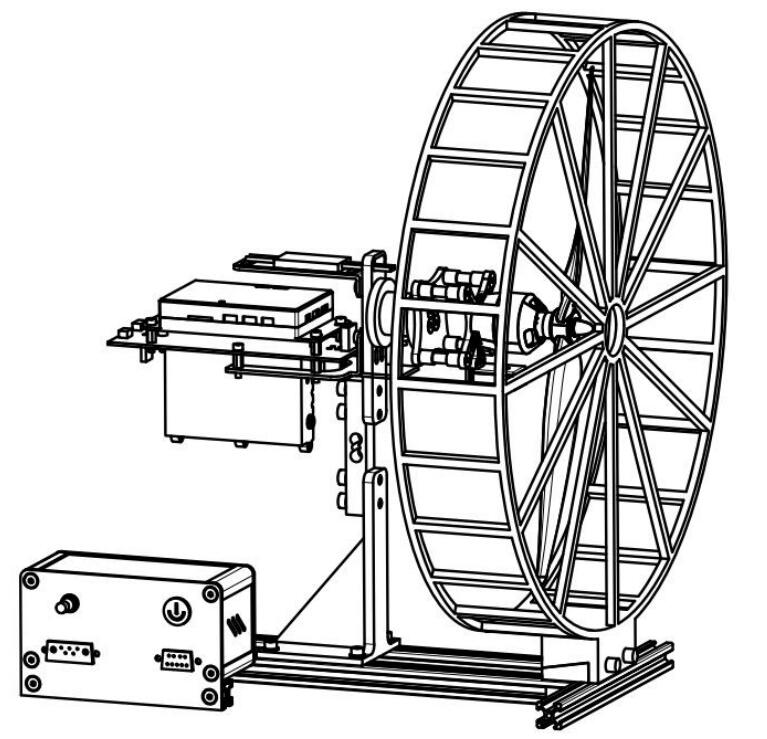## Course Content:

1. The introduction of performance parameters and performance characteristic curves of dynamic system

2. Test experiment of performance parameters and performance characteristic curves of dynamic system

## Experimental equipment:

WF-EDU-02 UAV dynamic test teaching instrument test kit

## Experimental teaching Objectives:

1. Understand the dynamic system performance parameters and performance characteristic curve;

2. Understand the performance analysis and influence of dynamic system performance parameters and characteristic curves on UAV, dynamic system, motor and propeller;

3. Master the test method of dynamic system performance parameters and performance characteristic curve;

## I. Explanation of experimental teaching principle:

### 1. Performance parameters and performance characteristic curves of dynamic system

The seven performance parameters of the dynamic system are thrust, torque, total power, shaft power, motor efficiency, propeller force efficiency and system force efficiency. Thrust and torque are the basic test parameters of the test bench, and the other five performance parameters are derived from the calculation of the basic test parameters of the test bench.

In the process of UAV flight, the running state of UAV dynamic system changes constantly, so the performance of UAV dynamic system cannot be judged by simple or single performance parameters. The performance analysis of dynamic system is mainly based on the performance characteristic curve, which represents all operating conditions of dynamic system throttle value from 0 to 100%. The characteristic curve of dynamic system includes throttle characteristic curve and speed characteristic curve.

The abscissa of throttle characteristic curve is throttle, and the ordinate is the characteristic curve of dynamic system performance parameters. The speed of characteristic curve is the characteristic curve of the performance parameters of the dynamic system on the horizontal coordinate of the speed and the vertical coordinate of the dynamic system.

The throttle characteristic curve is mainly for the performance analysis of the whole dynamic system or the motor, among which the throttle tension curve, the throttle total power curve and the throttle system force effect characteristic curve are mainly used for the overall performance analysis of the dynamic system. Throttle -torque and throttle - motor efficiency are mainly used for motor performance analysis.

The speed characteristic curve is mainly aimed at the performance analysis of the propeller, mainly including the speed - tension, speed - torque, speed - propeller force effect characteristic curve.

### 1.1 Thrust and tension characteristic curve

Thrust is one of the most critical performance parameters to ensure the safety of UAV takeoff. The size of UAV thrust and throttle thrust characteristic curve are directly related to take-off weight, load,

maneuverability (vertical climbing speed, flight speed) and safety control margin. Thrust is produced by the propeller, so it is also one of the important performance parameters of the propeller. The rotational

speed - thrust characteristic curve can well reflect the thrust characteristics of the propeller.

Throttle thrust characteristic curve: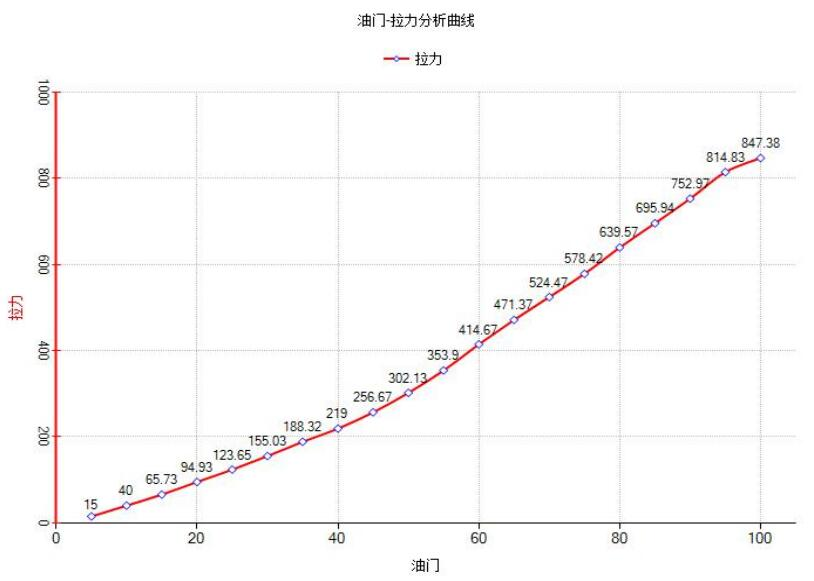Figure 2-1 Throttle - thrust characteristic curve

The throttle-thrust characteristic curve represents the thrust value corresponding to different throttle positions. Through the throttle-thrust curve, the throttle position corresponding to the hovering thrust can be accurately found, and based on which to judge whether the dynamic system thrust is suitable for the take-off weight of UAV.

A. Generally, the nominal voltage of the battery is used to test whether 40%-60% of the throttle thrust meets the hovering thrust, which is the first safety factor for UAV flying under the current take-off weight. (According to different throttle - thrust characteristic curves of dynamic system or different design requirements of UAV, the percentage of thrust throttle satisfying hover will be selected differently. For example,

the hover throttle of the traverser may be less than 40%.)

B. The take-off weight of UAV must be within a certain safety factor, and the general safety factor should be 70% of the thrust under the state of lowest electric quantity (empirical value), that is, 70% throttle thrust under the state of low battery voltage should not be less than the thrust required by UAV hovering.

Rotational speed thrust characteristic curve:Figure 2-2 Rotational speed - thrust characteristic curve

The rotational speed - thrust characteristic curve represents the thrust value produced by the propeller at different rotational speeds. The rotational speed - thrust curve can be compared and analyzed for propellers with different parameters or contours.

### 2.2 Torque and torque characteristic curve

The torque parameter, namely the counter-torque of the propeller, represents the air resistance generated by the rotation of the propeller, and the rotational speed - torque characteristic curve represents the air resistance characteristics of the propeller at different rotational speeds. The reverse torque generated by the propeller is balanced by the torque generated by the motor, so the torque represents the load of the

motor, and the throttle-torque curve reflects the power output performance of the motor.

Rotational speed and torque characteristic curve: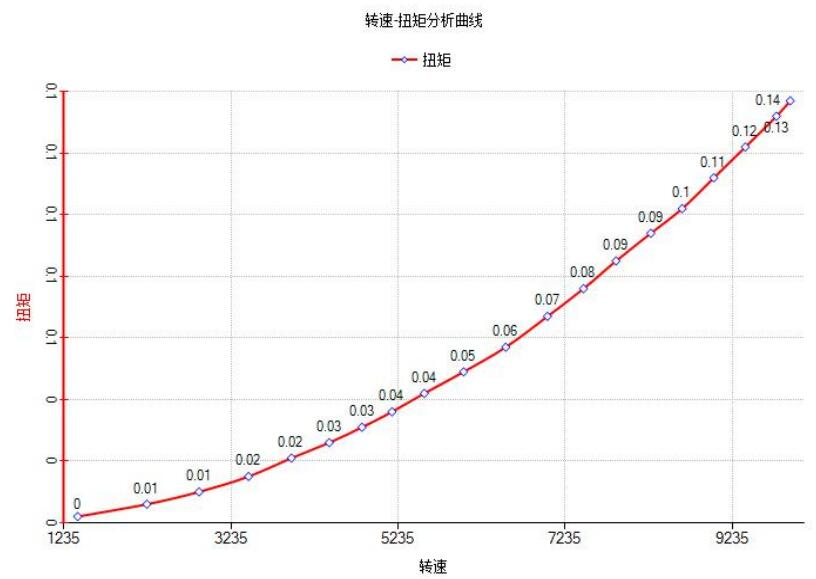Figure 2-3 Rotational speed - torque characteristic curve

Throttle torque characteristic curve:Figure 2-4 Throttle - torque characteristic curve

### 1.3 The Total Power

Total power is the total power consumed by UAV dynamic during operation, including the power of propeller driven by motor and thermal energy consumption of power system (thermal effect of current). It is an important performance index of UAV flight calculation, and the calculation formula is as follows:

PTotal = Uout * ITRMS                      (W)

Refers to the input voltage of the dynamic system, that is, the battery voltage, the unit is V; U out ITRMS Refers to the current of the power system, and the single operating current level is A. The hovering power and cruising power of dynamic system are one of the important parameters used to evaluate the aeroplane performance.

Throttle - total power characteristic curve: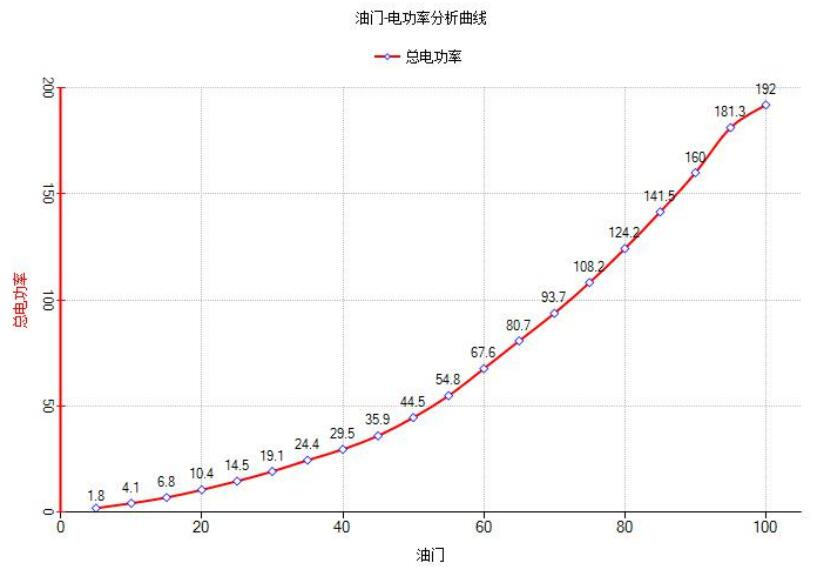Figure 2-5 Throttle - total power characteristic curve

The throttle-total power characteristic curve represents the total power consumption of the corresponding power system at different throttle positions. The corresponding power consumption value can be found on the curve according to the hovering throttle or cruising throttle, and the endurance of the UAV in hovering or cruising state can be calculated on this basis.

### 1.4 shaft power

Shaft power is the dynamic part of the motor in the dynamic system to convert electrical power into mechanical energy, also known as the output power of the motor, which refers to the power generated when the motor drives the propeller to rotate to overcome the air resistance. The calculation formula is:

Paxis = n*M             (W)

Where, is the rotational speed of the propeller, calculated in unit, and refers to the counter torque generated by the propeller, in unit. n rad / s M N · m The concept of speed and torque were mentioned in the previous section, and the shaft power of the motor is the product of the two.

Throttle shaft power characteristic curve: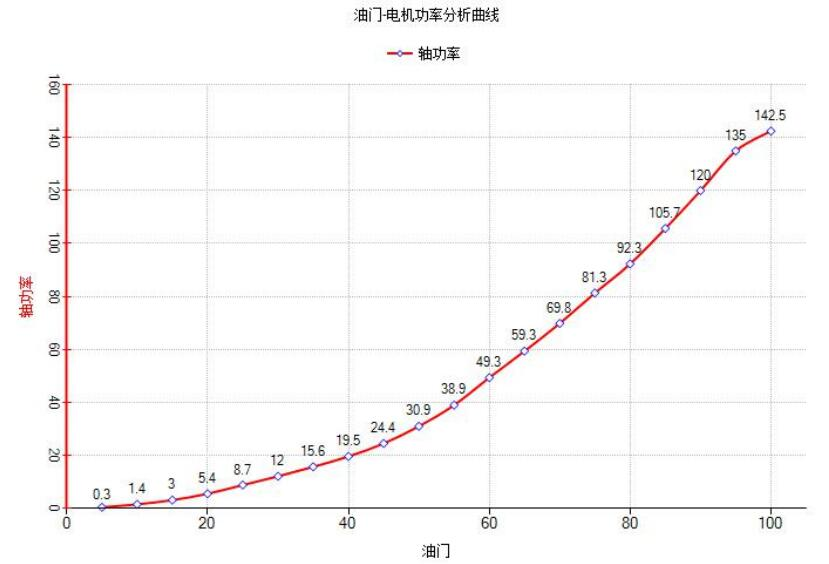Figure 2-6 Throttle - shaft power characteristic curve

The throttle - shaft ratio characteristic curve represents the shaft power of the motor corresponding to different throttle positions, reflecting the power output performance of the motor.

### 1.5 Motor efficiency

Motor efficiency is the efficiency of electrical energy into mechanical energy to do external work. Motor efficiency mainly depends on the design level of the motor, manufacturing technology, material selection and other factors, so the motor efficiency directly reflects the advantages and disadvantages of motor efficiency performance.

The total power includes the power that the motor drives the propeller to rotate and do work (shaft power) and the power consumption of the dynamic system (the thermal effect of the current). The components of the heat consumption mainly include the stator winding of the electric harmonic motor. The electric modulation is the control unit of the motor, so the heat consumption is counted as a part of the motor. The motor efficiency is the proportion of the shaft power of the motor in the total power, and the formula is: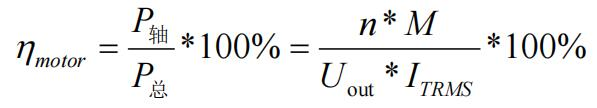Throttle motor efficiency curve: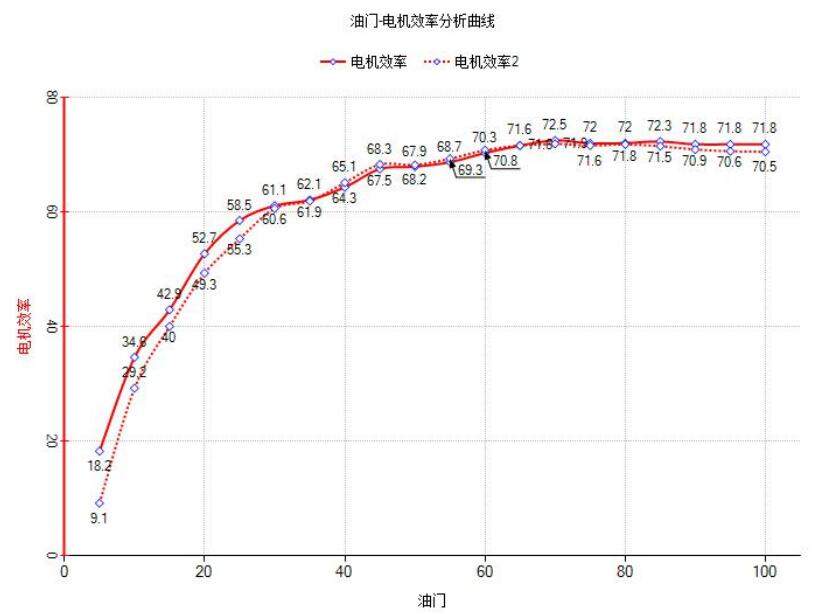Figure 2-7 Throttle motor efficiency characteristic curve

The throttle motor efficiency characteristic curve can optimize the selection of different motors under the condition of determining the load. For a motor, when the load changes, the throttle motor efficiency characteristic curve of the motor will have a great impact, so the motor selection cannot be completely determined by the throttle motor efficiency characteristic curve.

### 1.6 Propeller force efficiency

Propeller force efficiency represents the comprehensive efficiency of the rotor propeller. The actual meaning of force efficiency is how many grams of thrust can be generated per 1W power, in unit of g/W. Here, power refers to the power required to drive the propeller to rotate and offset the reverse torque of the propeller, namely the shaft power of the motor. The calculation formula of propeller force effect is as

follows: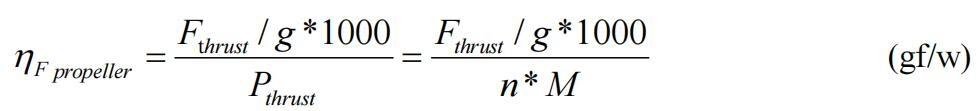Note: The concept of "force efficiency" is an efficiency concept proposed on the basis of rotor-craft UAV. Under the original fixed wing calculation formula, the efficiency of the rotor-craft propeller would be calculated as 0, so the concept of "force efficiency" is proposed.

Force effect characteristic curve of rotating speed and propeller: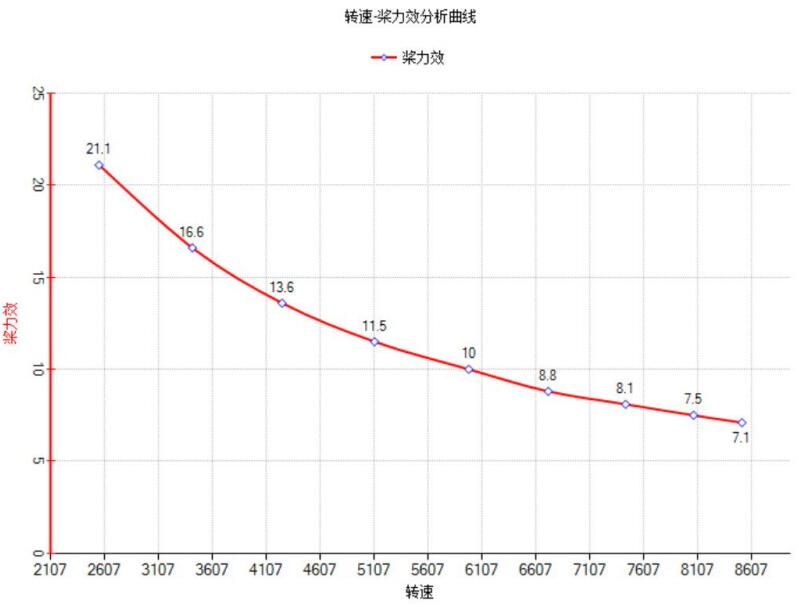Figure 2-8 Speed - propeller force - effect characteristic curve

Speed-propeller force-effect characteristic curve directly embodies the rotor efficiency of the propeller performance, but when the propeller performance analysis, usually combined with a speed - pulling force characteristic curve of the propeller performance comprehensive judgment, not only through the speed - comprehensive performance of propeller propeller force effect judgment, to complete the

optimization of propeller type selection. The optimal selection of propeller is mainly based on the pull-propeller force - effect characteristic curve.

### 1.8 System force efficiency

System force effect (G /W) refers to the thrust generated by every 1W power of the total power of the dynamic system. It is the most intuitive parameter of the flight efficiency of the whole UAV and an important parameter that directly reflects the power consumption and efficiency of the UAV. Its calculation formula is as follows: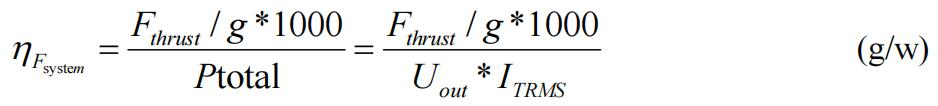The relationship between motor efficiency, propeller force efficiency and system force efficiency is as follows: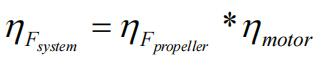Therefore, the system force efficiency includes motor efficiency and propeller force efficiency, reflecting the overall efficiency of UAV.

Throttle - system force effect curve: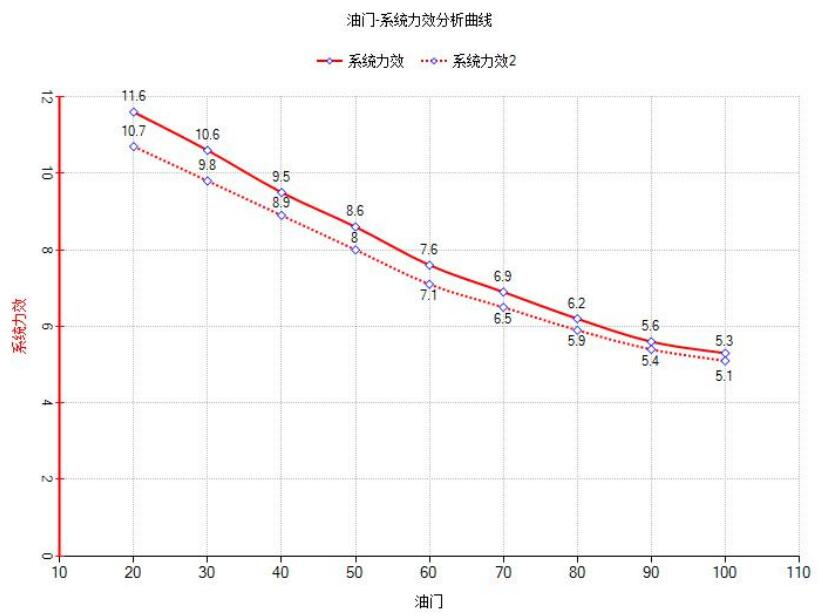Figure 2-10 Throttle - system force - effect characteristic curve

Two groups of matched power systems can directly select the optimal power system selection and matching scheme of UAV through throttle system force effect characteristic curve analysis.E-mail: sandy@wing-flying.com

Add.: 7th Floor, B2#，Animation Building, Sino-Singapore Tianjin Eco-City